1603955695

# Introduction to Graph Neural Networks

Graph neural networks — their need, real-world applications, and basic architecture with the NetworkX library

In this post, we are going to investigate a relatively newer field in deep learning which involves graphs — a very important and widely used data structure. This post encompasses the basics of graphs, the amalgamation of graphs and deep learning, and a basic idea about graph neural networks and their applications. We will also briefly discuss on how to build graphs with a Python library called NetworkX

So, let’s dive right in!

## What are graphs?

In the world of computer science, graphs are a type of data structure having two components: Nodes (or vertices) and edges, which connect two nodes. Thus, a graph can be defined as a collection of loosely inter-connected nodes via edges.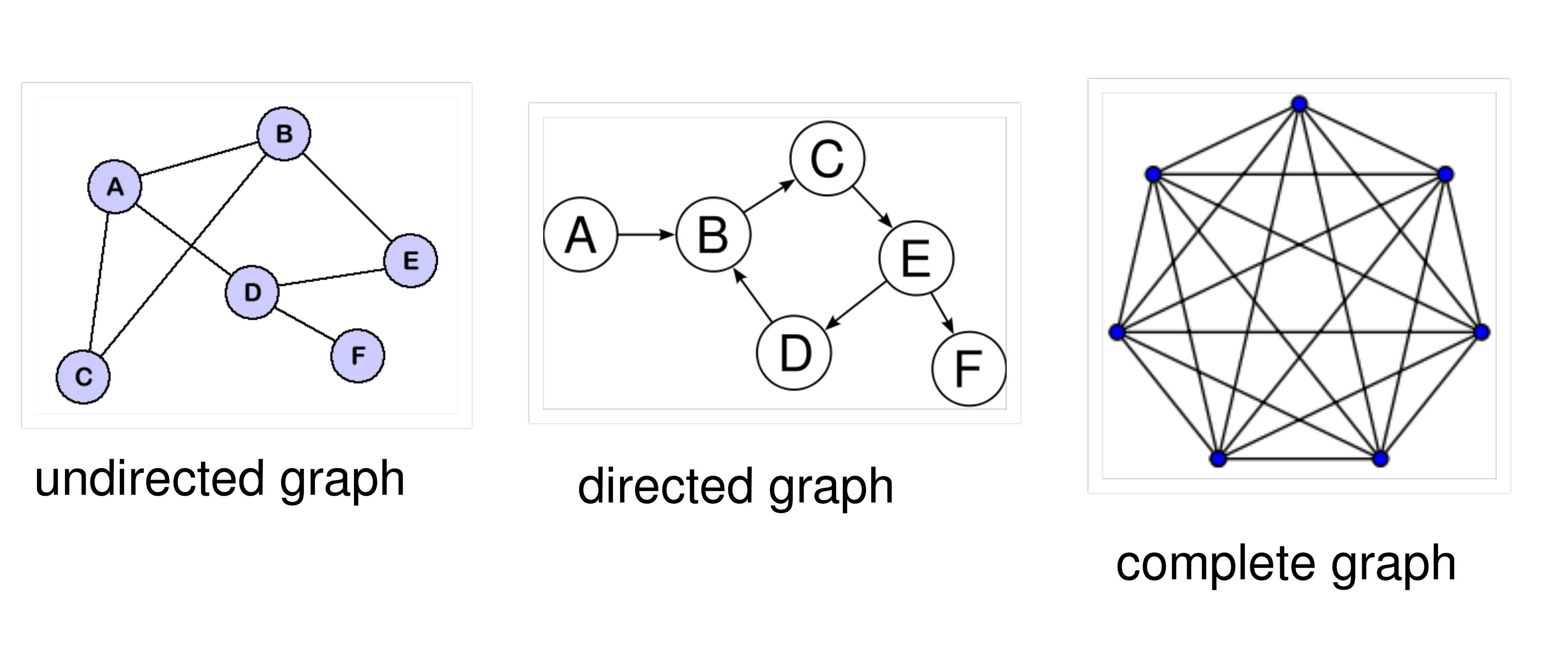Thenodes of a graph can be homogenous with all nodes having a similar structure, or heterogenous nodes having different types of structure. The edges define the relationship one node has with another. Edges can be bidirectional (from one node u to another v and vice versa), or unidirectional (from one node u to another node v). Edges can also be weighted — having a weight assigned to the edge that might depict the edge’s cost or importance.

An example: Let us suppose a graph to be considered as a network of cities — the cities under observation being nodes and the roads connecting them being edges. Now, there can be various types of relevant problems that can be solved with graphs, such as finding out the shortest distance between cities (where roads can also be weighted as per the condition of the roads or traffic), or finding the cities which are well-connected to each other, etc.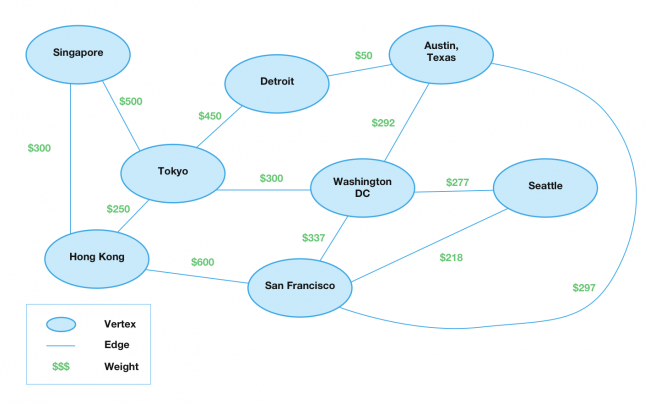### What are Graph Neural Networks (GNN)?

Graphs have tremendous expressive powers and are therefore gaining a lot of attention in the field of machine learning. Every node has an embedding associated with it that defines the node in the data space. Graph neural networks refer to the neural network architectures that operate on a graph. The aim of a GNN is for each node in the graph to learn an embedding containing information about its neighborhood (nodes directly connected to the target node via edges). This embedding can then be used for different problems like node labelling, node prediction, edge prediction, etc.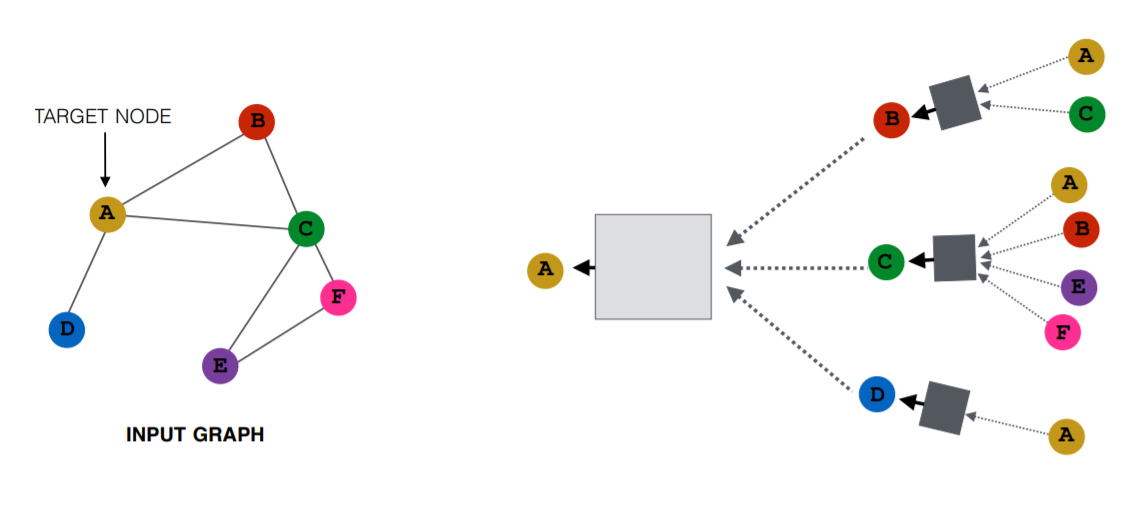Each node and its neighborhood

Thus, after having embeddings associated with each node, we can convert edges by adding feed forward neural network layers and combine graphs and neural networks.

### Need for Graph Neural Networks

The need for graph neural networks arose from the fact that a lot of data available to us is in an unstructured format. Unstructured data is data that has not been processed or does not have a pre-defined format which makes it difficult to analyze. Examples of such data are audio, emails, and social media postings. To make sense of this data and to derive inferences from it, we need a structure that defines a relationship between these unstructured data points. The existing machine learning architectures and algorithms do not seem to perform well with these kinds of data. The primary advantages of graph neural networks are:

1. The graph data structure has proven tremendously successful in the field of computer science while working with unstructured data.
2. Graphs are helpful in defining concepts which are abstract, like relationships between entities. Since each node in the graph is defined by its connections and neighbors, graph neural networks can capture the relationships between nodes in an efficient manner.

Thus, developing GNNs for handling data like social network data, which is highly unstructured, is an exciting amalgamation of graphs and machine learning which holds a lot of potential.

### Real-Life Applications of Graph Neural Network

Being introduced recently in 2018, the GNNs still have a lot of real-life applications because their architecture resonates with the irregularity in data collected from various sources. Currently, GNNs have been the hot topic for:

Social Network Analysis — Similar posts prediction, tags prediction, and recommending content to users.

Natural Sciences — GNNs have also gained popularity in dealing with molecular interactions like protein-protein interactions.

Recommender Systems — A heterogenous graph can be used to capture relationships between users and items to recommend relevant items to a buyer.

#machine-learning #python #data-science #developer

## Buddha Community1623135499

## No Code introduction to Neural Networks

### The simple architecture explained

Neural networks have been around for a long time, being developed in the 1960s as a way to simulate neural activity for the development of artificial intelligence systems. However, since then they have developed into a useful analytical tool often used in replace of, or in conjunction with, standard statistical models such as regression or classification as they can be used to predict or more a specific output. The main difference, and advantage, in this regard is that neural networks make no initial assumptions as to the form of the relationship or distribution that underlies the data, meaning they can be more flexible and capture non-standard and non-linear relationships between input and output variables, making them incredibly valuable in todays data rich environment.

In this sense, their use has took over the past decade or so, with the fall in costs and increase in ability of general computing power, the rise of large datasets allowing these models to be trained, and the development of frameworks such as TensforFlow and Keras that have allowed people with sufficient hardware (in some cases this is no longer even an requirement through cloud computing), the correct data and an understanding of a given coding language to implement them. This article therefore seeks to be provide a no code introduction to their architecture and how they work so that their implementation and benefits can be better understood.

Firstly, the way these models work is that there is an input layer, one or more hidden layers and an output layer, each of which are connected by layers of synaptic weights¹. The input layer (X) is used to take in scaled values of the input, usually within a standardised range of 0–1. The hidden layers (Z) are then used to define the relationship between the input and output using weights and activation functions. The output layer (Y) then transforms the results from the hidden layers into the predicted values, often also scaled to be within 0–1. The synaptic weights (W) connecting these layers are used in model training to determine the weights assigned to each input and prediction in order to get the best model fit. Visually, this is represented as:

#machine-learning #python #neural-networks #tensorflow #neural-network-algorithm #no code introduction to neural networks1595691960

## How Graph Convolutional Networks (GCN) Work

In this post, we’re gonna take a close look at one of the well-known Graph neural networks named GCN. First, we’ll get the intuition to see how it works, then we’ll go deeper into the maths behind it.

## Why Graphs?

Many problems are graphs in true nature. In our world, we see many data are graphs, such as molecules, social networks, and paper citations networks.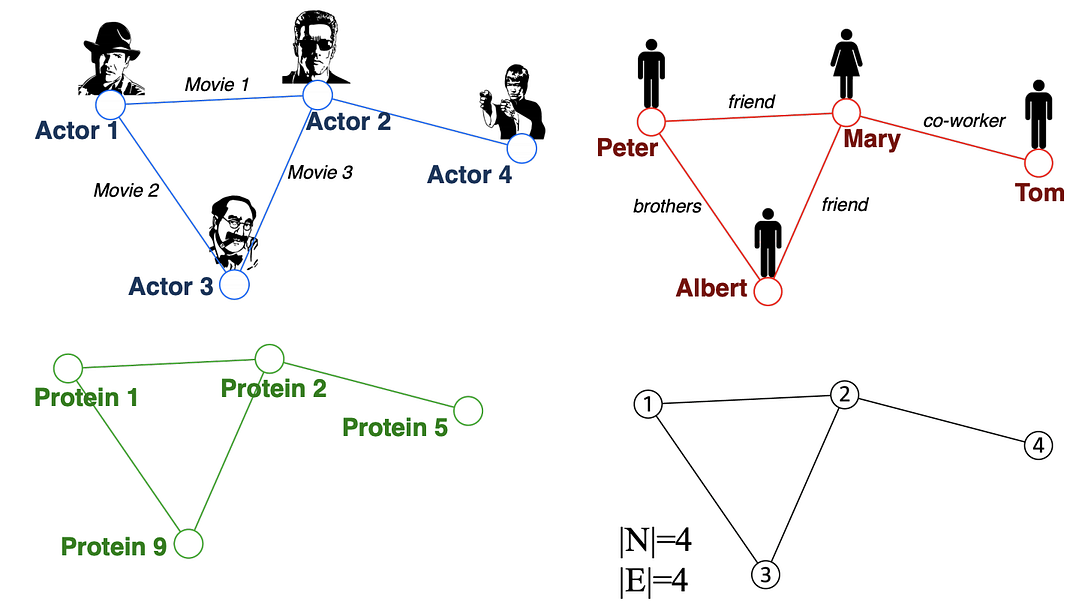Examples of graphs. (Picture from )

• Node classification: Predict a type of a given node
• Community detection: Identify densely linked clusters of nodes
• Network similarity: How similar are two (sub)networks

## Machine Learning Lifecycle

In the graph, we have node features (the data of nodes) and the structure of the graph (how nodes are connected).

For the former, we can easily get the data from each node. But when it comes to the structure, it is not trivial to extract useful information from it. For example, if 2 nodes are close to one another, should we treat them differently to other pairs? How about high and low degree nodes? In fact, each specific task can consume a lot of time and effort just for Feature Engineering, i.e., to distill the structure into our features.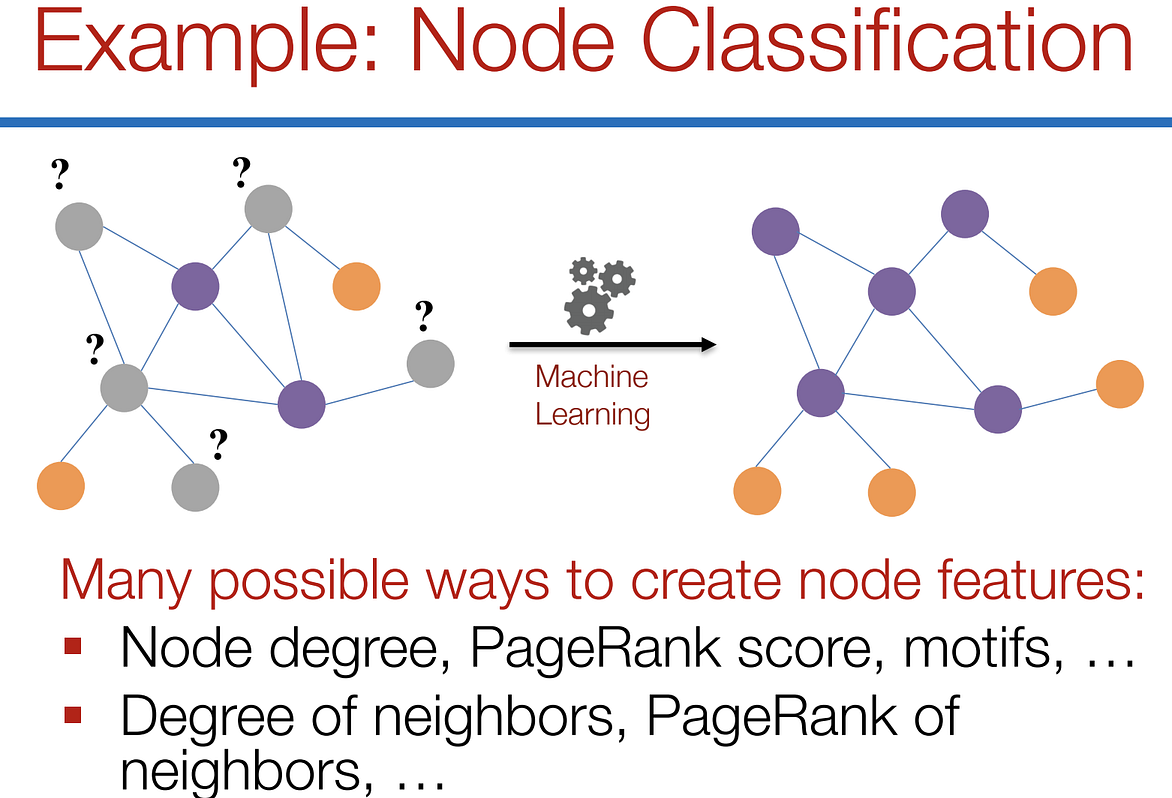Feature engineering on graphs. (Picture from )

It would be much better to somehow get both the node features and the structure as the input, and let the machine to figure out what information is useful by itself.

That’s why we need Graph Representation Learning.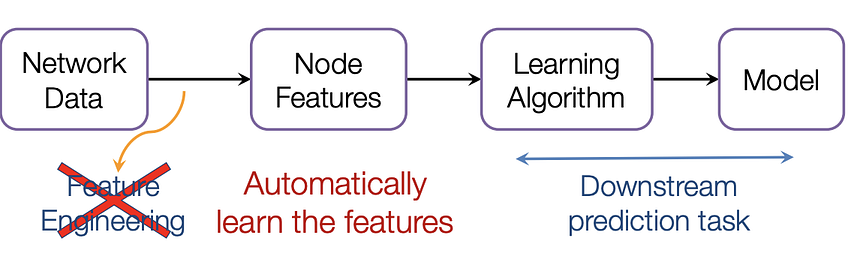We want the graph can learn the “feature engineering” by itself. (Picture from )

# Graph Convolutional Networks (GCNs)

Paper: Semi-supervised Classification with Graph Convolutional Networks(2017) 

GCN is a type of convolutional neural network that can work directly on graphs and take advantage of their structural information.

it solves the problem of classifying nodes (such as documents) in a graph (such as a citation network), where labels are only available for a small subset of nodes (semi-supervised learning).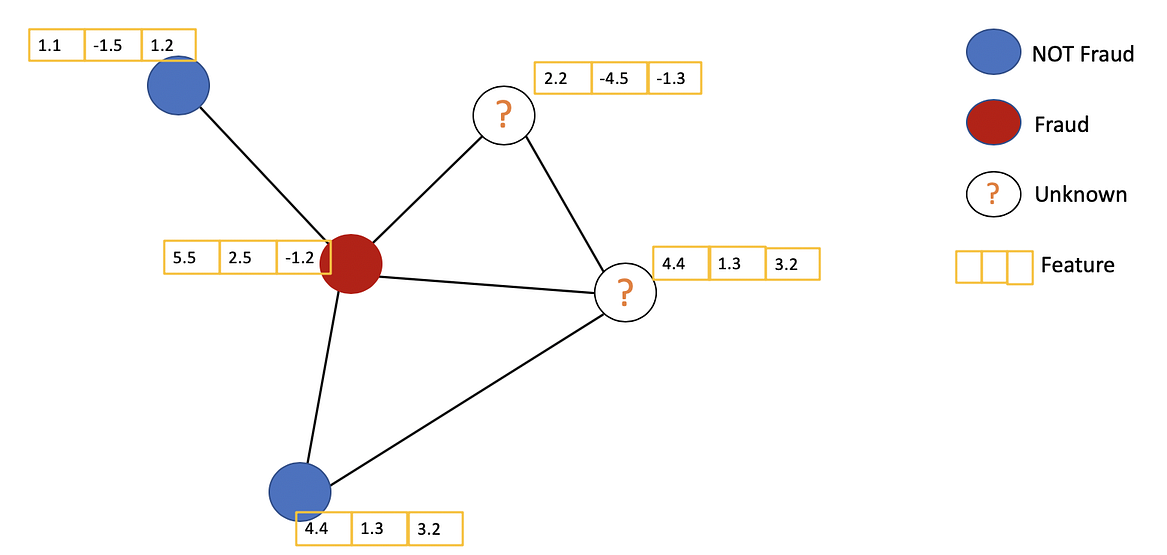Example of Semi-supervised learning on Graphs. Some nodes dont have labels (unknown nodes).

#graph-neural-networks #graph-convolution-network #deep-learning #neural-networks1602838800

## SimGNN: Similarity Computation via Graph Neural Networks

This post will summarize the paper SimGNN which aims for fast graph similarity computation. Graphs are structures that are used to link different entities that we call nodes using relationships called edges. Graphs exist everywhere from bonds between the atoms to friends on Facebook, all these scenarios can be represented as a graph. One of the fundamental graph problems includes finding similarity between graphs. The similarity between graphs can be defined using these metrics :

1. Graph Edit Distance
2. Maximum Common Subgraph

However, currently available algorithms that are used to calculate these metrics have high complexities and it is not yet possible to compute exact GED using these for graphs having more than 16 nodes.

Some ways to compute these metrics are :

1. Pruning verification Framework
2. Approximating the GED in fast and heuristic ways

SimGNN follows another approach to tackle this problem i.e turning similarity computation problem into a learning problem.

Before getting into how SimGNN works, we must know the requirements to be satisfied by this model. It includes :

1. Representation Invariant: Different representations of the same graph should give the same results.
2. **Inductive: **Should be able to predict results for unseen graphs.
3. Learnable: Must work on different similarity metrics like GED and MCS

**SimGNN Approach: **To achieve the above-stated requirements, SimGNN uses two strategies

1. Design Learnable Embedding Function: This maps the graph into an embedding vector, which provides a global summary of a graph. Here, some nodes of importance are selected and used for embedding computation. (less time complexity)
2. Pair-wise node comparison: The above embedding are too coarse, thus further compute the pairwise similarity scores between nodes from the two graphs, from which the histogram features are extracted and combined with the graph level information. (this is a time-consuming strategy)

#graph-edit-distance #machine-learning #graph-neural-networks #graph-convolution-network1594478340

## How to Explain Graph Neural Network — GNNExplainer

Graph Neural Network(GNN) is a type of neural network that can be directly applied to graph-structured data. My previous post gave a brief introduction on GNN. Readers may be directed to this post for more details.

Many research works have shown GNN’s power for understanding graphs, but the way how and why GNN works still remains a mystery for most people. Unlike CNN, where we can extract activation of each layer to visualize the decisions of the network, in GNN it is hard to get a meaningful explanation of what features the network has learnt. Why does GNN determine a node is class A instead of class B? Why does GNN determine a graph is a chemical or molecule? It seems like GNN sees some useful structural information and determines are made upon these observations. But now the problem is, what observations does GNN see?

## What is GNNExplainer?

GNNExplainer is introduced in this paper.

Briefly speaking, it is trying to build a network to learn what a GNN has learnt.

The main principle of GNNExplainer is by reducing redundant information in a graph which does not directly impact the decisions. To explain a graph, we want to know what are the crucial features or structures in the graph that affect the decisions of a neural network. If a feature is important, then the prediction should be altered largely by removing or replacing this feature with something else. On the other hand, if removing or altering a feature does not affect the prediction outcome, the feature is considered not essential and thus should not be included in the explanation for a graph.

## How does it work?

The primary objective for GNNExplainer is to generate a minimal graph that explains the decision for a node or a graph. To achieve this goal, the problem can be defined as finding a subgraph in the computation graph, that minimizes the difference in the prediction scores using the whole computation graph and the minimal graph. In the paper, this process is formulated as maximizing the mutual information(MI) between the minimal graph Gs and the computation graph G: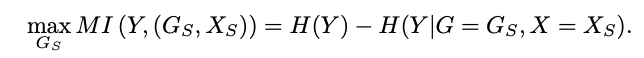Besides, there is a secondary objective: the graph needs to be minimal. Though it was also mentioned in the first objective, we need to have a method to formulate this objective as well. The paper addresses it by adding a loss for the number of edges. Therefore, the loss for GNNExplainer is literally the combination of prediction loss and edge size loss.

#graph #graph-neural-networks #graph-theory #pattern-recognition #machine-learning1594474140

## Benchmarking Graph Neural Networks

Graph Neural Networks (GNNs) are widely used today in diverse applications of social sciences, knowledge graphs, chemistry, physics, neuroscience, etc., and accordingly there has been a great surge of interest and growth in the number of papers in the literature.

However, it has been increasingly difficult to gauge the effectiveness of new models and validate new ideas that generalize universally to larger and complex datasets in the absence of a standard and widely-adopted benchmark.

To address this paramount concern existing in graph learning research, we develop an open-source, easy-to-use and reproducible benchmarking framework with a rigorous experimental protocol that is representative of the categorical advances in GNNs.

This post outlines the issues in the GNN literature suggesting the need of a benchmark, the framework proposed in the paper, the broad classes of widely used and powerful GNNs benchmarked and the insights learnt from the extensive experiments._

# Why benchmark?

In any core research or application area in deep learning, a benchmark helps to identify and quantify what types of architecturesprinciples, or mechanisms are universal and generalizable to real-world tasks and large datasets. Particularly, the recent revolution in this AI field is often credited, to a possibly large extent, to be triggered by the large-scale benchmark image dataset, ImageNet. (Obviously, other driving factors include increase in the volume of research, more datasets, compute, wide-adoptance, etc.)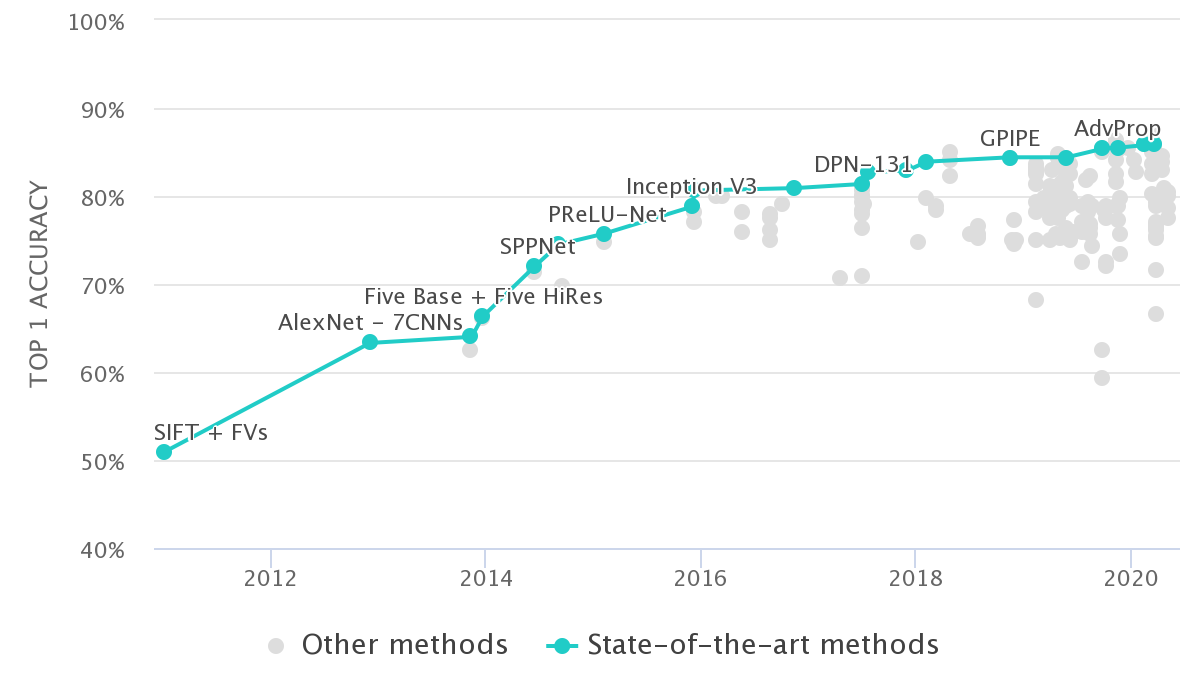Fig 1: ImageNet Classification Leaderboard from paperswithcode.com

Benchmarking has been proved to be beneficial for driving progress, identifying essential ideas, and solving domain-related problems in many sub-fields of science. This project was conceived with this fundamental motivation.

# Need of a benchmarking framework for GNNs

## a. Datasets:

Many of the widely cited papers in the GNN literature contain experiments that are evaluated on small graph datasets which have only a few hundreds (or, thousand) of graphs.Fig 2: Statistics of the widely used TU datasets. Source Errica et al., 2020

Take for example, the ENZYMES dataset, which is almost seen in every work on a GNN for classification task. If one uses a random 10-fold cross validation (in most papers), the test set would have 60 graphs (i.e. 10% of 600 total graphs). That would mean a correct classification (or, alternatively a misclassification) would change 1.67% of test accuracy score. A couple of samples could determine a 3.33% difference in performance measure, which is usually a significant gain score stated when one validates a new idea in literature. You see there, the number of samples is unreliable to concretely acknowledge the advances.¹

Our experiments, too, show that the standard deviation of performance on such datasets is large, making it difficult to make substantial conclusions on a research idea. Moreover, most GNNs perform statistically the same on these datasets. The quality of these datasets also leads one to question if you should use them while validating ideas on GNNs. On several of these datasets, simpler models, sometimes, perform as good, or even beats GNNs.

Consequently, it has become difficult to differentiate complexsimple and graph-agnostic architectures for graph machine learning.

## b. Consistent experimental protocol:

Several papers in the GNN literature do not have consensus on a unifying and robust experimental setting which leads to discussing the inconsistencies and re-evaluating several papers’ experiments.

For a couple of examples to highlight here, Ying et al., 2018 performed training on 10-fold split data for a fixed number of epochs and reported the performance of the epoch which has the “highest average validation accuracy across the splits at any epoch” whereas Lee et al., 2019 used an “early stopping criterion” by monitoring the epoch-wise validation loss and report “average test accuracy at last epoch” over 10-fold split.

Now, if we extract results of both these papers to put together in the same table and claim that the model with the highest performance score is the promising of all, can we get convinced that the comparison is fair?

There are other issues related to hyperparamter selection, comparison in an unfair budgets of trainable parameters, use of different train-validation-test splits, etc.

The existence of such problems pushed us to develop a GNN benchmarking framework which standardizes GNN research and help researchers make more meaningful advances.

#benchmarking #graph #graph-deep-learning #graph-neural-networks #deep-learning